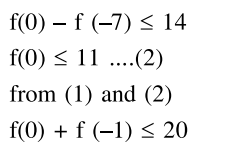Deepak Scored 45->99%ile with Bounce Back Crack Course. You can do it too!

# Let the function,

Question:

Let the function, $f:[-7,0] \rightarrow \mathrm{R}$ be continuous on $[-7,0]$ and differentiable on $(-7,0)$. If $f(-7)=-3$ and $f^{\prime}(\mathrm{x}) \leq 2$, for all $\mathrm{x} \in(-7,0)$, then for all such functions $f, f(-1)+f(0)$ lies in the interval:

1. $[-6,20]$

2. $(-\infty, 20]$

3. $(-\infty, 11]$

4. $[-3,11]$

Correct Option: , 2

Solution:

Using LMVT in $[-7,-1]$

$\frac{f(-1)-f(-7)}{-1-(-7)} \leq 2$

$f(-1)-f(-7) \leq 12$

$\Rightarrow \mathrm{f}(-1) \leq 9 \ldots .(1)$

Using LMVT in $[-7,0]$

$\frac{f(0)-f(-7)}{0-(-7)} \leq 2$